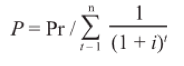# Calculating an Amortized PaymentMarch 30, 2017

When you have a loan where the payment is a fixed amount, to be divided on a monthly (or other periodic) basis between interest and principal, how do you calculate the correct payment that will cause that loan to pay off in the specified term? The classical equation for this case is shown below:In the above equation, P is the payment. Pr is the total principal amount. n is the number of payments. i is the periodic interest rate. Because the interest rate is periodic and n is the total number of payments, and therefore the total number or periods, the actual period and the actual length of the term do not need to be defined.

The Greek letter Sigma signifies a summation. The equation 1/(1+i)^t is added together for each successive instance of t=1 through t=n. While this is easy to do (if n is a small number) on a spread sheet, it is not so easy to program as an equation. If you integrate this as a series integration (that's second year calculus), you end up with an actual equation without a summation:

P = Pr x ((i/(1-(1+i)^(-n))))

Using calculus, we have reduced the problem to one of straight algebra, which is an improvement. However, there are a couple of things that this standard form equation, notwithstanding which of these two forms we take it in does not take into account.

1. Periodic interest: This periodic interest rate assumes that each payment period is being handled as if it was of equal length. If the payment period is monthly and the interest rate is 12%, then i = 1%. That means that the interest is 1% in January, but it is also 1% in February. This is important because each day in January is 1/31 of 1% and each day in February is 1/28 of 1% which is a higher rate. What if we are using one of the interest year calculations where every day of the year is 1/365 of 12%? In this case, we have i for the month of January equal to 12% x 31/365 = 1.01917808% and i for the month of February = 12% x 28/365 = 0.9205479452%. The net result of this is that we would end up with a different payment amount on the same loan if the loan originated on the January 1 vs February 1. It is a not insignificant effect.

2. Irregular first period 1. What if the loan originates on January 15 and the first payment is not until March 1. There is no way to do a summation where n is a fraction (although it is technically possible to put a fraction for n in the equation derived from the summation). In any case, those extra days will have an effect, and there is no easy equation that will account for both of these things.

So, how do we do it?

The best thing to do is to use a really strongly featured amortization schedule calculator that can project the loan forward with the hypothetical assumption of all payments being made on their due dates and then look at the end of that schedule to see how far off we are on the final payment. Then we will adjust the payment amount until the amount off on the final payment reaches a minimum value.

I will run through an example of how this is done.

Let's assume a loan of 12 monthly payments with the origination date on January 15 and the first payment date on March 1. The interest rate is 12%, and the principal amount is \$10,000.

By the above equations, the monthly payment amount is \$888.49.

If we were to run an amortization schedule, we would find that our final payment is \$945.73. This deviates from the rest of the payments by \$57.24. We take this number and divide it by the total number of payments (12) to get \$4.77. We add that to the payment amount (\$888.49) to get \$893.26. Now if we do a payment override to that amount and run the amort schedule again, the final payment on that amort schedule is \$889.98. This deviates from the payment we now have by -\$3.28. Dividing that by the number of payments, we get -0.27, and adding that to the payment amount gives \$892.99. Once again we use this as a payment override and try the amort schedule. Final payment is \$893.14. The difference is \$0.15. Dividing by 12 we get one cent. So we try \$893.00. The final payment on the amort schedule comes out as 893.02. The difference of two cents, when divided by the number of payments yields zero. No change. \$893.00 is the answer.

In the Nortridge Loan System, there is a function that will automatically run through this exact procedure. In the loan setup, set your amort method to "Amortize Based on Actual Interest Year."

The payment given by the loan system in this case is precisely \$893.00, which we know to be the best fit payment for this situation.

And that (to quote a famous astronaut) is how we do that.